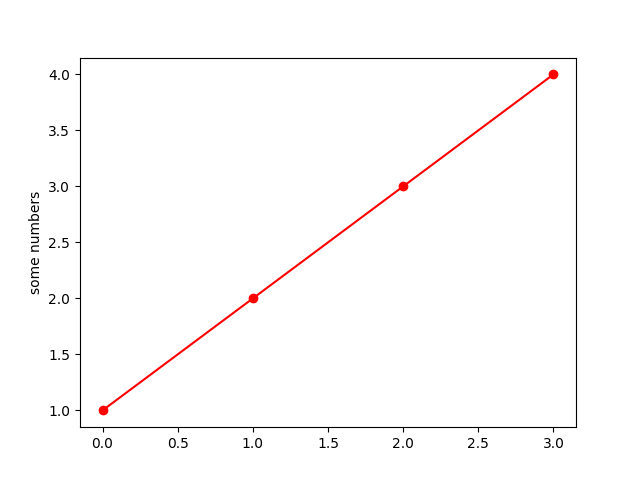# Simple plot#

A simple plot where a list of numbers are plotted against their index, resulting in a straight line. Use a format string (here, 'o-r') to set the markers (circles), linestyle (solid line) and color (red).

import matplotlib.pyplot as plt

plt.plot([1, 2, 3, 4], 'o-r')
plt.ylabel('some numbers')
plt.show()References

The use of the following functions, methods, classes and modules is shown in this example:

Gallery generated by Sphinx-Gallery